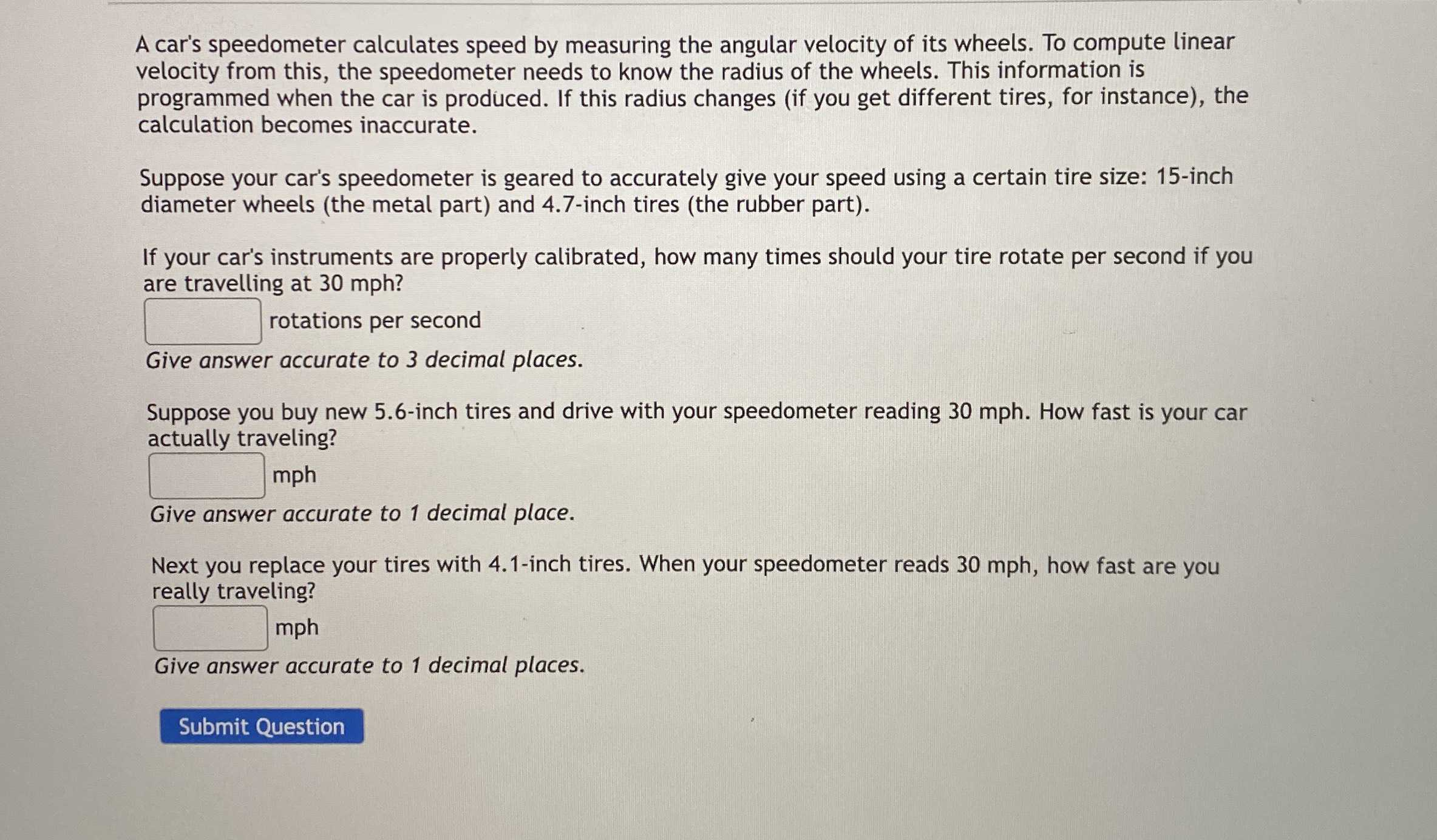### ¿Todavía tienes preguntas de matemáticas?

Pregunte a nuestros tutores expertos
Algebra
PreguntaA car's speedometer calculates speed by measuring the angular velocity of its wheels. To compute linear velocity from this, the speedometer needs to know the radius of the wheels. This information is programmed when the car is produced. If this radius changes (if you get different tires, for instance), the calculation becomes inaccurate.

Suppose your car's speedometer is geared to accurately give your speed using a certain tire size: 15-inch diameter wheels (the metal part) and $$4.7$$ -inch tires (the rubber part).

If your car's instruments are properly calibrated, how many times should your tire rotate per second if you are travelling at $$30$$ mph?

Give answer accurate to $$3$$ decimal places.

Suppose you buy new $$5.6$$ -inch tires and drive with your speedometer reading $$30$$ mph. How fast is your car actually traveling?

Give answer accurate to $$1$$ decimal place.

Next you replace your tires with $$4.1$$ -inch tires. When your speedometer reads $$30$$ mph, how fast are you really traveling? Give answer accurate to $$1$$